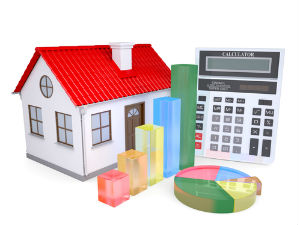# How is interest and principal divided in an EMI?

|
Looking to take a loan? It's extremely important to understand the concept of how your equated monthly installment is divided between your interest and principal repayment.

Let's take a simple example. Say you take a loan of Rs 2 lakhs for years at an interest rate of 18 per cent per annum. The total amount you would be paying each month is Rs 7230, which for 36 months works out to Rs 260,280. Which means that for borrowing Rs 2 lakhs, you are going to pay back Rs 2,60,280.

Now, each month you pay Rs 7,230, which has some interest and some principal amount. In the initial years the break-up would have a significant amount of interest. In the first month the interest payment would be Rs 3000 and Rs 4230 would be the principal amount.

Interest Payment Principal Payment

Month 1 Rs 3000 Rs 4200

Month 2 Rs 2936 Rs 4294

Month 6 Rs 2673 Rs 4557

Month 12 Rs 2247 Rs 4983

Month 15 Rs 2012 Rs 5211

In the above example, you can see in the initial years, you are paying a significant amount towards interest and hardly repaying the principal amount. After a few months the principal amount will start increasing and the interest reducing.

Therefore, its important to remember that if you want to pay back your loan after a few months, the amount left to be paid back would be substantial given that in the initial years you have been repaying only the interest.

How is EMI calculated?

EMI comprises of two variable components those are principal amount and interest rate. The component of interest amount is higher in initial years and decreases over the years. EMI is calculated using the factors like interest rates, loan amount and the tenure of the repayment.read How is EMI calculated

GoodReturns.in